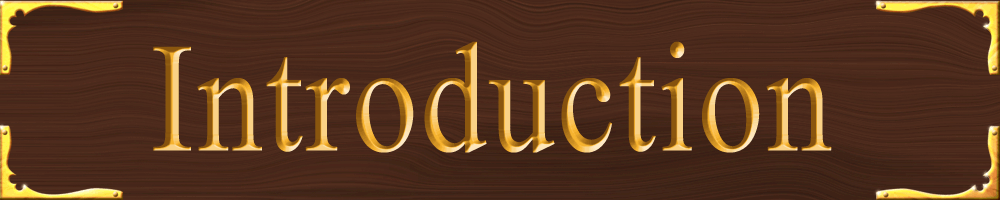## Why even study Physics at all?

Physics is the study of how things work. But you can’t think of it that way. Science- ALL of Science- is a study of Nature. It’s sometimes hard to make the connection between clouds and grass and ice cream and music and art with equations and chemicals- but yes. It is the study of Nature.

The unfortunate thing about learning the way of Nature is that Science is broken down into major disciplines so that you can focus on one thing at a time. Nature is not. The true epiphany is to realize that each subject does not stand alone- “hey now I’m gonna do some Chemistry, then I’m gonna do some Physics.” No! They all happen at the same time.

My doctor made me promise to give a message to my students: “You must realize that all Sciences work together. When I have to replace a patient’s bladder, I am not just doing Medicine, not just Biology. I also am doing Math to figure out the volume of the organ. I am doing Physics to make sure that the new organ will be strong enough and work right. I am doing Chemistry as I use medicines in the right amounts for the patient. And I am doing all these things at once and on the fly. I cannot make detailed measurements and then do calculations before I open the patient. I have to do it all right there, right then. All of it at the same time. In addition, I am doing Art when I shape the organ.

You can be a victim of Nature or you can be a Partner with it. To let the world just happen to you is to be a victim. There is an amazing amount of pleasure that comes from looking around the world and having some understanding of what’s really going on. And just as much pleasure to know what is not understood. To look at the world around you with understanding and awe, as Master of all and also to be humbled by it is to be a partner with Nature.

• The Metric System (MKS system) has seven fundamental units. They are
• Meter : distance
• Kilogram : mass (roughly “how many atoms make up an object”)
• Second : time
• Ampere: electric current (how many atoms are flowing)
• Mole : the number of atoms needed to equal the atomic mass number in grams (1 mole of Carbon has a mass of 12 g and contains 6.022…×1023 atoms)
• Kelvin : temperature
• Candela : light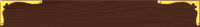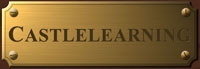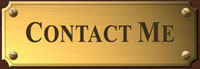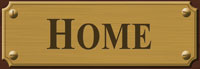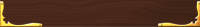## Worksheets

Lab Reports Format

Approximations Lab

Estimations

Interpreting Graphs• Prefixes are also used to increase or decrease the size of any unit.
• Some Common Prefixes
• Tera- T 1012 “trillion” (Terabyte computer hard drive)
• Giga- G 109 “billion” (1.21 Gigawatts of energy to power the flux capacitor)
• Mega- M 106 “million” (Mega! Big! M! Million!)
• Kilo- K 103 “thousand” (a kilometer is like a metric mile, 1,000 meters like 1,000yards)
• centi- c 10-2 “one hundredth” (100 cents in a dollar ∴ one cent is 1/100th of a dollar)
• milli- m 10-3 “one thousandth” (a millipede has, like, a thousand legs)
• micro- µ (mu) 10-6 “one millionth” (million and micro both start with “m”)
• nano- n 10-9 “one billionth” (nono- nino- nine zeroes)
• All physical measurements are subject to error. Errors may stem from the method used, environmental fluctuations, instrument limitations, or personal error.
• Systematic errors are in one direction only.
• Random errors are in both directions and may be reduced by large numbers of observations (repeated trials).
• Tolerance is the amount of accepted error. It leads to a Range of acceptable values.
• The accuracy of a measurement refers to how closely it agrees with an accepted value. (AKA percent deviation or percent error)
• Precision is the degree of exactness of a measurement and is determined by the instrument used.
• For handheld instruments the precision is half the smallest mark (which is estimated).
• Significant figures are those known to be reasonably reliable. Therefore all measured figures and one estimated figure would be considered significant.
• Digits 1 to 9 are always significant. Zeroes are significant when they appear between digits; if they follow digits a decimal must be present in the number for the zeroes to be significant.
• Addition and Subtraction: When adding or subtracting numbers, your answer will have the same number of significant figures after the decimal as the least precise number used.
• For example: 25.678 + 3.45 + 67.2 = 96.3
• Only to the tenths place
• Multiplication and Division: When multiplying or dividing numbers, your answer cannot have more total significant figures than the smallest total number of significant figures in any of the numbers used to obtain the answer.
• For example: (26.4 N)(1.2 m) = 32 N•m Only two significant figures
• These rules are fairly easy to follow until you begin introducing zeros into your equations. Below are some examples using zeros.
• 700 has only one significant figure (the 7).
• 700.0 has 4 significant figures (all 4 numbers).
• 0.0700 has 3 significant figures (the 7 and the two zeros to the right of the 7).
• 0.007 has only 1 significant figure (the number 7).
• 7.007 has 4 significant figures (all 4 numbers).
• For very large and very small quantities we write numbers in scientific notation.

## Greek Alphabet- used as variables or symbols

Many letters represent specific values or measurements

 Use in Physics Capital Name Lower Case Use in Physics Α Alpha α angle Β Beta β angle torque Γ Gamma γ E/mc2, angle change Δ Delta δ small change Ε Epsilon ε electromotive force Ζ Zeta ζ Η Eta η index of refraction angle Θ Theta θ most common symbol for angle Ι Iota ι rarely used- looks too much like i Κ Kappa κ rate, lambda baryon Λ Lambda λ wavelength, rate Μ Mu μ coefficient of friction, muon, micro- Ν Nu ν frequency, nutrino charmed baryon Ξ Xi ξ Ο Omicron ο rarely used- looks like 0 product operator Π Pi π Hello! Pi! also a pion Ρ Rho ρ density, resistivity sum of Σ Sigma σ conductivity, cross section, area density Τ Tau τ mean lifetime, tau lepton Υ Upsilon υ rearely used- look slike v wave function, angle Φ Phi φ wave function, angle Χ Chi χ wave function Ψ Psi ψ wave function Ω Omega ω angular frequency

## Unit Conversions

• Before you solve an exercise, it is important that all units on the ends of the numbers you are using be in the same system. Most of the quantities you will see this year use the SI System (Systeme International), which is the standard system of units in physics.
• To convert units into an equivalent amount using different units you will need to multiply by fractions which equal 1 and cancel out like units.
• For example: To convert 5 years into seconds.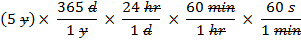• Notice that anything you are trying to eliminate in the numerator must be written in the denominator and vice versa.
• The units appearing in both the numerator and denominator cancel each other out, as shown by the slash marks through them.
• Multiplying the numerators gives: 5 x 365 x 24 x 60 x 60= 157,680,000
• Multiplying the denominators gives: 1 x 1 x 1 x 1=1
• Final answer: 157,680,000s/1 = 157,680,000 s. Note: This number is not written with significant figures.

## Dimensional Analysis

• All numbers in physics have units after the number.
• For example:
• 10
• 10 m, 10 s, 10 g, 10 peanut butter sandwiches
• The units desired will often lead you to the equation needed to do the math.
• For example:
• To get an answer of m/s 2 (acceleration) you will need to multiply meters by seconds 2 (seconds x seconds)
• By the way if “seconds squared” sounds too weird pronounce it as “meters per second per second.” Which means “for every second, your speed increases by so many meters per second”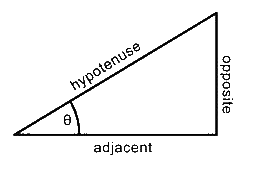## Some Simple Trigonometry Relationships

• The rules of trigonometry are developed with the use of right triangles as shown in the labeled diagram. Using this diagram, you can construct trigonometric equations in the following way.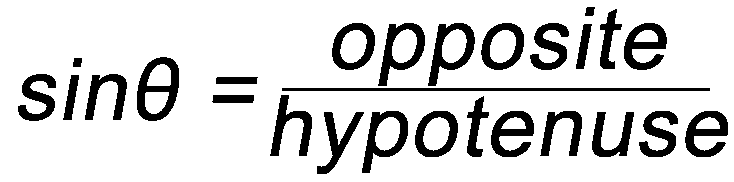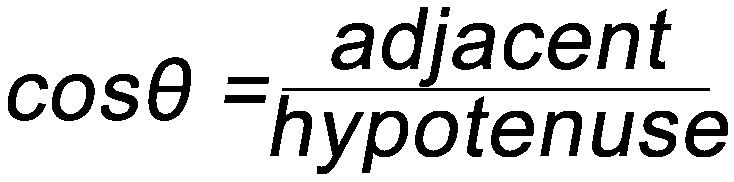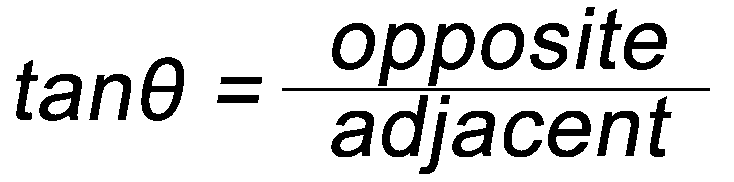• Remember, you can only use the above relationships with right triangles. The hypotenuse of a right triangle is always the longest side.Medina On-Line
Since 1994
Email: mrsciguy@optonline.net